#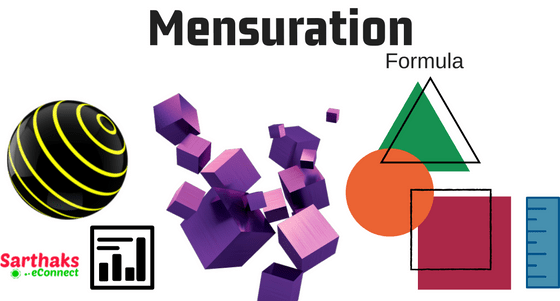## Mensuration Formula:

At first, let us know about Mensuration. It is a branch of mathematics which deals with the measurement of several parameters such as length, breadth, perimeter, area and volume of 2-dimensional and 3-dimensional objects. Mensuration formula for class 6, 7, 8, 9, 10 contains data of lines and angles for measurement of several parameters. Generally, it is used for geometrical figures where there is a need to find out dimensions, perimeter, area, volume and several other physical quantities.

Perimeter: It is the path surrounding the object’s area. We can also say that the length of boundary of an object is its perimeter. It is simply the addition of length so it has no powered units. Simply its unit is in m (metre), cm (centimetre), km (kilo-metre) etc. Mensuration formula for perimeter is different for different figures.

Area: It is the surface occupied by the 2-dimensional body. We can also define the area as that surface of object in a plane closed within a boundary. Millions of lines in a plane coexist to form an area so Its unit is squared as we multiply length to lengths. Its units are cm2, m2, km2 etc. The mensuration formula for area is different for different geometrical bodies.

Volume: It is the space occupied by any three-dimensional body within its surface boundary. How much Space it occupies includes several dimensions such as length, breadth, height. So its unit is always expressed in cubic units as cm3, m3, km3etc.

## List of all the necessary mensuration formula orderly:

2D figures Figures Formula

## Mensuration formula for TrianglePerimeter= Sum of all sidesArea = 1/2 b x h

Area = $$\sqrt{s(s-a)(s-b)(s-c)}$$

a, b, c are sides of triangle.

Where s=$$\frac{a+b+c}{2}$$

Area=$$\frac{1}{2}$$ (bxh)

## Mensuration formula for Equilateral TrianglePerimeter= 3xSide

Area = $$\frac{\sqrt{3}}{4}$$ (side)2

## Mensuration formula for SquarePerimeter: 4 x side

Area: (side)2

## Mensuration formula for RectanglePerimeter= 2(length + breadth)

## Mensuration formula for Parallelogram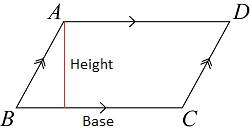Perimeter= 2(1st side + 2nd side)

Area= base x height

## Mensuration formula for RhombusPerimeter= 4 x side

Area= $$\frac{1}{2}$$ D1 x D2

## Mensuration formula for TrapeziumPerimeter= sum of all sides

Area= $$\frac{1}{2}$$ (sum of parallel sides)x altitude

## Mensuration formula for Circle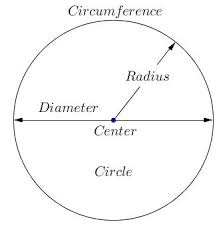Circumference= 2πr

Area= πr2 (where r is radius)

## Mensuration formula for Semicircle

Area=$$\frac{1}{2}$$πr2

## Mensuration formula for 3-D figures:

3 D figures  Figures Formula

## Mensuration formula for Cuboid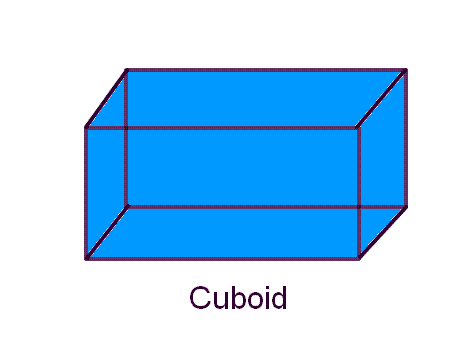Surface Area: 2(lb+bh+lh)

Volume = L x B x H

Diagonal = $$\sqrt{l^{2}+b^{2}+h^{2}}$$

## Mensuration formula for Cube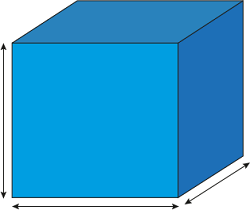Surface Area= 6 x (side)2

Volume = (side)3

Diagonal = $$\sqrt{3}$$a

## Mensuration formula forRight prism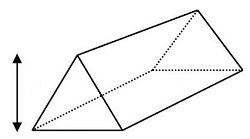Lateral or curved surface area = perimeter of base x height

Total surface area =  lateral surface area + 2(area of ends)

Volume= Area of base x Height

## Mensuration formula for Right Circular cylinderLateral surface area = 2πrh

Total surface area= 2πrh + 2πr2

Volume= πr2h

## Mensuration formula for Pyramid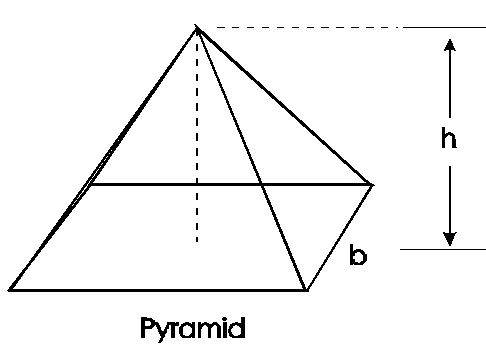Lateral surface area= $$\frac{1}{2}$$ perimeter of base x slant height

Total surface area= lateral surface area + area of base

Volume= $$\frac{1}{3}$$ (area of base) x height

## Mensuration formula for Sphere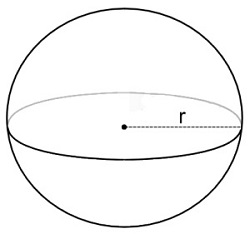Surface area= 4πr2

Volume= $$\frac{4}{3}$$πr3

## Mensuration formula for HemisphereLateral Surface area= 2πr2

Total surface area= 3πr2

Volume= $$\frac{2}{3}$$πr3

## Mensuration formula for Right circular cone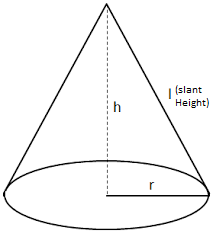Slant Height= l = $$\sqrt{r^{2}+h^{2}}$$

Lateral surface area= πrl

Total surface area = πrl + πr2

Volume= $$\frac{1}{3}$$ πr2h

## Mensuration formula for Frustum of a coneformulas Slant Height= l = $$\sqrt{{h}^{2}+ ({r}_1-{r}_2)^{2}}$$

Lateral surface area= πl(r1+r2)

Total surface area= πl(r1+r2) + πr12+ πr22

Volume= $$\frac{1}{3}$$πh(r12+r1r2+r22)

## Mensuration formula for Bank, railway, SSC exam and for class 6th to 10th:

The list of formulas with well-defined images will help you to memorise it easily. The formula list will help you to solve the problems related to mensuration much easily. Basic formulas are for class 6, then a little more added in each section of class 7, class 8, class 9, class 10 and so on.

## FAQ on Mensuration Formula:

1.  What are mensuration formulas?
Mensuration itself means To measure. In mathematics, these formulas help in a different kind of measurement of 2D, 3D figure such as its perimeter, lateral and total surface area, Volume. These formula ease the problem-solving way.
2. How to remember mensuration formulas?
To remember mensuration formulas you must make application of each formula in different types of problems. The more you practice problems more you will learn. Here formulas with figures is given so that it will be easy for you to learn.
 More Formulas Triangle Formulas Circle Formulas Lines and Angles Formula Algebra Formulanew Number system Calculus formulas